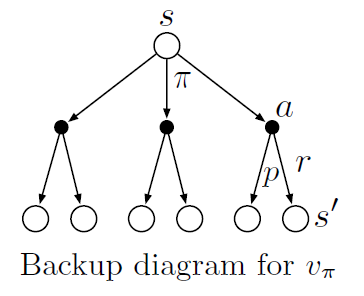# Chapter 3 Finite Markov Decision Processes

Different from multi-armed brandit problem, MDPs have an associative aspect - choosing different actions in different situations.

## 3.1 The Agent-Environment Interface

Agent: the learner and decision maker.

Environment: what the agent interact with, or anything that cannot be changed by the agent.

Definition of agent-environment interaction: At each given time step $t$, the agent receives the environment's state $S_t\in\mathcal{S}$, and on that basis selects an action, $A_t\in\mathcal{A}(s)$. Then the agent receives a numerical reward, $R_{n+1}\in \mathcal{R} \subset\mathbb{R}$, and find itself in a new state of $S_{t+1}$

Finite MDP: the set of states, actions, rewards all have a finite number of elements.

The dynamics of the MDP:

$$p(s^\prime, r | s, a) \doteq \text{Pr} [ S_t=s^\prime, R_t=r | S_{t-1}=s, A_{t-1}=a ]$$ for all $s^\prime, s\in\mathcal{S}, r\in\mathcal{R}, a\in\mathcal{A}(s)$.

And we can denote the function as $\mathcal{S}\times\mathcal{R}\times\mathcal{S}\times\mathcal{A}\rightarrow[0,1]$

MDP is defined in a way that $S_t$ and $R_t$ only depends on $S_{t-1}$ and $R_{t-1}$. Thus, the state is required to include past agent-environment interaction information, and this is called Markov property.

State-Transition Probabilities:

This is a three-argument function: $\mathcal{S}\times\mathcal{S}\times\mathcal{A}\rightarrow[0,1]$

$$p(s^\prime|s, a) \doteq \text{Pr}[S_t=s^\prime|S_{t-1}=s, A_{t-1}=a]=\sum_{r\in\mathcal{R}}{p(s^\prime, r | s, a) }$$

The expected rewards for state-action pairs, a two-argument function $r: \mathcal{S}\times\mathcal{A}\rightarrow\mathcal{R}$

$$r(s,a) \doteq \mathbb{E}[R_t|S_{t-1}=s,A_{t-1}=a]=\sum_{r\in\mathcal{R}}{r\sum_{s^\prime\in\mathcal{S}}{p(s^\prime,r|s,a)}}$$

The expected rewards for state-action-next-state triple as a three-argument function $r: \mathcal{S}\times\mathcal{A}\times\mathcal{S}\rightarrow\mathcal{R}$ (compared to the last scenario, we now know the state after the action) $$r(s,a,s^\prime) \doteq \mathbb{E}[R_t|S_{t-1}=s,A_{t-1}=a,S_t=s^\prime]=\sum_{r\in\mathcal{R}}{\frac{p(s^\prime,r|s,a)}{p(s^\prime|s,a)}}$$

The boundary of agent and environment: agent's absolute control.

## 3.2 Goals and Rewards

Goal: to maximize cumulative reward.

The reward signal should be what you want the robot to achieve, not how you want it to achieve.

## 3.3 Returns and Episodes

Goal: maximize expected return.

Return, denoted by $G_t$, is defined as some specific function of the reward sequence, the simplest case the return would be:

$$G_t=R_{t+1}+R_{t+2}+R_{t+3}+...+R_{T}$$

Episodes: the subsequences of agent-environment interaction, end at the terminal state.

Terminal state: the last state $T$.

• Nonterminal states: $\mathcal{S+}$

• All states: $\mathcal{S}$

Return for continuing tasks - disconted return

$$G_t\doteq R_{t+1}+\gamma R_{t+2}+\gamma^2 R_{t+3}+...=\sum_{k=0}^\infty\gamma^kR_{t+k+1}$$

Where $\gamma\in[0,1]$, called discount rate.

$$G_t\doteq R_{t+1}+\gamma G_{t+1}$$

## 3.4 Unified Notation for Episodic and Continuing Tasks

$S_{t,i}$: State $S$ at time step $t$ and episode $i$. But we usually use $S_t$ when $i$ is not important.

Absorbing state: a state only transitions to itself and generates only rewards of zero.

Unified notation:

$$G_t \doteq \sum^T_{k=t+1}{\gamma}^{k-t-1}R_k$$

Including the possibility that $T=\infty$ or $\gamma=1$ but not both.

## 3.5 Policies and Value Functions

$\pi(a|s)$: the probability that $A_t=a$ if $S_t=s$.

Value functions: functions of states (or state-action pairs) that estimate how good it is for the agent to be in a given state. "How good" means expected return. It is the expected return when starting in $s$ and following $\pi$ thereafter.

State-value function for policy $\pi$:

$$v_\pi(s) \doteq \mathbb{E}_\pi[G_t|S_t=s] = \mathbb{E}_\pi\left[ \sum_{k=0}^{\infty}{\gamma^kR_{t+k+1}}\middle | S_t=s \right]\text{, for all }s\in S$$

Action-value function for policy $\pi$:

$$q_\pi(s,a) \doteq \mathbb{E}_\pi[G_t|S_t=s,A_t=a] = \mathbb{E}_\pi\left[ \sum_{k=0}^{\infty}{\gamma^kR_{t+k+1}}\middle | S_t=s, A_t=a \right]$$

Policies: particular ways of acting, a mapping form states to probabilities of selecting each possible action.

Monte Carlo methods: estimate $q_\pi$ and $v_\pi$, we keep averages for each state and each action. Or, we can also set $q_\pi$ and $v_\pi$ as parameterized functions.

The recursive relationships of value function (Bellman Equation):

$$v_\pi(s) \doteq \mathbb{E}[G_t|S_t=s] = \mathbb{E}_\pi[R_{t+1}+\gamma G_{t+1}|S_t=s]$$

$$\Downarrow$$

$$v_\pi(s) = \sum_a{\pi(a|s)\sum_{s^\prime,r}{p(s^\prime,r|s,a)\left[r+\gamma v_\pi(s^\prime) \right]}} \text{ (Bellman Equation for } v_\pi\text{)}$$

Understanding: State → action made by policy → get state and reward → new state

Backup diagrams## 3.6 Optimal Policies and Optimal Value Functions

Evaluation of policy: $\pi\geq\pi^\prime\iff v_\pi(s)\geq v^\prime_{\pi'}(s)$ for all $s$.

Optimal policy: $\pi_\ast$

Optimal state-value function: $v_\ast(s)\doteq \max_\pi v_\pi(s)$ for all $s\in\mathcal{S}$

Optimal action-value function: $q_\ast(s,a)\doteq \max_\pi q_\pi(s,a)$ for all $s\in\mathcal{S}$ and $a\in\mathcal{A}$

Relation between $q_\ast$ and $v_\ast$ $$q_\ast(s,a) = \mathbb{E}[R_{t+1}+\gamma v_\ast(S_{t+1})|S_t=s,A_t=a]$$

Bellman optimality equation $$v_\ast(s) = \max_a q_{\pi_\ast}(s,a)$$ $$v_\ast(s) = \max_a{\mathbb{E}}[R_{t+1}+\gamma v_\ast(S_{t+1}|S_t=s,A_t=a)]$$ $$v_\ast(s) = \max_a{\sum_{s',r}p(s',r|s,a)[r+\gamma v_\ast(s')]}$$

For finite MDP, the Bellman optimality equation has one unique solution. If there are $n$ states, then this are $n$ equations in $n$ unknowns.